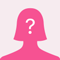# HELP ME TO SOLVE THIS MATH QUESTION, PLEASE?Can you help me with these math questions and provide solution? Thanks

1. If the average speed of an MRT train is 90kph, find the time it takes to travel from one station to another if the distance between the station is 6km.

2. An express train leaves one station at 6:18am and arrive at the next station at 6:24am. Find the average speed of the train, in kph. If the distance traveled is 8.4km.

3. A motorist averaged 75kph in going a distance of 300 km. He made the return trip over the same distance in 6 hours. Find his average speed for the entire trip.

4. If 2x + y + z = 21 and x + y + z = 9, then x = ?

5. If x is 150% of y, then y is what percent of x + y?
HELP ME TO SOLVE THIS MATH QUESTION, PLEASE?
2 Opinion